# Sound

## Exercise 7(A)

#### Question 1

What are mechanical waves?

A mechanical wave is a wave that is not capable of transmitting it's energy through vacuum. They require a medium in order to transport their energy from one location to another.

Example — Sound wave.

The mechanical waves are of two types —

(a) Longitudinal waves and

(b) Transverse waves.

#### Question 2

Define the following terms in relation to a wave — (a) amplitude, (b) frequency, (c) wavelength and (d) wave velocity.

(a) Amplitude (a) — When sound waves travel in a medium, the maximum displacement of the particle of medium on either side of its mean position, is called amplitude of the wave.

(b) Frequency (f) — The number of vibrations made by the particle of the medium in one second.

(c) Wavelength (λ) — The distance travelled by a wave in one time period of vibration of the particle of the medium, is called the wavelength.

(d) Wave velocity (V) — The distance travelled by the wave in one second.

#### Question 3

A wave passes from one medium to another medium. Mention one property of the wave out of speed, frequency or wavelength (i) which changes, (ii) which does not change.

(i) When a wave passes from one medium to another medium, the wavelength (or speed) of the wave changes.

(ii) When a wave passes from one medium to another medium, , the frequency of the wave does not change.

#### Question 4

State two factors on which the speed of a wave travelling in a medium depends.

The two factors on which the speed of a wave travelling in a medium depends are —

(i) Elasticity and

(ii) Density

#### Question 5

State two differences between the light and sound waves.

Light wavesSound waves
These are the electromagnetic waves.These are the mechanical waves.
They can travel in a vacuum.They require a material medium for propagation. They cannot travel in a vacuum.

#### Question 6

What do you mean by reflection of sound? State one condition for the reflection of a sound wave. Name a device in which reflection of a sound wave is used.

The return of a sound wave on striking a surface such as a wall, metal sheet, plywood etc. back in the same medium is called reflection of the sound wave.

The only requirement for the reflection of sound wave is that the size of the reflecting surface must be bigger than the wavelength of the sound wave.

The phenomenon of reflection of sound wave is utilized in making the megaphone (or speaking tube).

#### Question 7

What is meant by an echo? State two conditions necessary for an echo to be heard distinctly.

The sound heard after reflection from a distant obstacle (such as a cliff, a hillside, the wall of a building, edge of a forest, etc.) after the original sound has ceased, is called an echo.

The two conditions for an echo to be heard distinctly are —

1. The minimum distance between the source of sound (or observer) and the reflector in the air must be 17 m.
2. The size of the reflector must be large enough as compared to the wavelength of the sound wave

#### Question 8

A man is standing at a distance of 12 m from a cliff. Will he be able to hear a clear echo of his sound ? Give a reason for your answer.

As we know,

$\text{Speed of sound (V)} = \dfrac{\text{Total distance travelled (2d)}}{\text {time interval (t)}}$

Total distance travelled by the sound in going and then coming back = 2d

Given,

d = 12 m

V = 340 ms-1

Substituting the values in the formula above, we get,

$340 = \dfrac{2 \times 12}{t} \\[0.5em] t = \dfrac{2 \times 12}{340} \\[0.5em] t = 0.07 s \\[0.5em]$

Hence, time = 0.07 s.

An important condition for hearing an echo is that the reflected sound should reach the ear only after a lapse of at least 0.1 s after the original sound dies off.

The time in this case is less than 0.1 s. Hence, the man will not hear the echo.

#### Question 9

State two applications of echo.

The applications of echo are —

1. Dolphins detect their enemy and obstacle by emitting the ultrasonic waves and hearing their echo.
2. In medical field, echo method of ultrasonic wave is used for imaging the human organs (such as liver, gall bladder, uterus, womb, etc.) This is called ultrasonography.

#### Question 10

Explain how the speed of sound can be determined by the method of echo?

The echo method can be used to determine the speed of sound in air.

For this, sound is produced from a place at a known distance say, d at least 50 m from the reflecting surface. The time interval t in which the echo reaches the place from where the sound was produced, is noted by a stop watch having the least count 0.01 s . Then the speed is calculated by using the following relation —

V = $\dfrac{\text {Total distance travelled}}{\text{Time interval}}$

V = $\dfrac{\text {2d}}{\text {t}}$ ms-1

The experiment is repeated several times and then the average value of speed of sound V is determined.

#### Question 11

State the use of echo by a bat, dolphin and fisherman.

Bats fly with speed much lower than the speed of sound. The sounds produced by the flying bats gets reflected back from an obstacle in front of it. By hearing the echo, the bats come to know, even in the dark, the location of the obstacle, so they turn from their path and fly safely without colliding with it. This process of detecting obstacle is called sound ranging.

Dolphins detect their enemy and obstacle by emitting the ultrasonic waves and hearing their echo. They use ultrasonic waves for hunting their prey.

A fisherman sends an ultrasonic pulse (a very high frequency vibrator) from a source and receives the pulse reflected from the shoal of fish in a detector. Hence, a fisherman use echoes to catch fishes.

The total time (t) of the two and fro journey of the pulse is recorded. The distance d of fish is then calculated by using the relation —

$\text{d} = \dfrac{Vt}{2} \\[0.5em]$

where, V is nearly 1400 ms-1 (the speed of ultrasonic waves in sea water).

#### Question 12

How do bats avoid obstacles in their way, when in flight?

Bats fly with speed much lower than the speed of sound. The sounds produced by the flying bats gets reflected back from an obstacle in front of it. By hearing the echo, the bats come to know, even in the dark, the location of the obstacle, so they turn from their path and fly safely without colliding with it. This process of detecting obstacle is called sound ranging.

Bats can produce and detect the sound of very high frequency up to about 100 kHz.

#### Question 13

What is meant by sound ranging? Give one use of sound ranging.

Sound ranging is the process of detecting obstacles with the help of echo. Animals like bats, dolphin use sound ranging to detect their enemies.

#### Question 14

Name the waves used for sound ranging. State one reason for their use. Why are the waves mentioned by you not audible to us?

Ultrasonic waves are used for sound ranging.

Ultrasonic waves can travel undeviated through a long distance and so they are used for sound ranging.

The range of audible sound waves is 20 Hz to 20,000 Hz, whereas ultrasonic waves have a frequency more than 20,000 Hz. Hence, ultrasonic waves are not audible to humans.

#### Question 15

What is 'SONAR'? State the principle on which it is based.

The word 'SONAR' stands for Sound navigation and ranging.

The figure given below shows the principle of a SONAR in which, ultrasonic waves are sent in sea water in all directions, from the ship. These waves are received after reflection from an obstacle such as the enemy submarine, iceberg, sunken ship etc. Hence, the distance between the ship and the obstacle can be determined.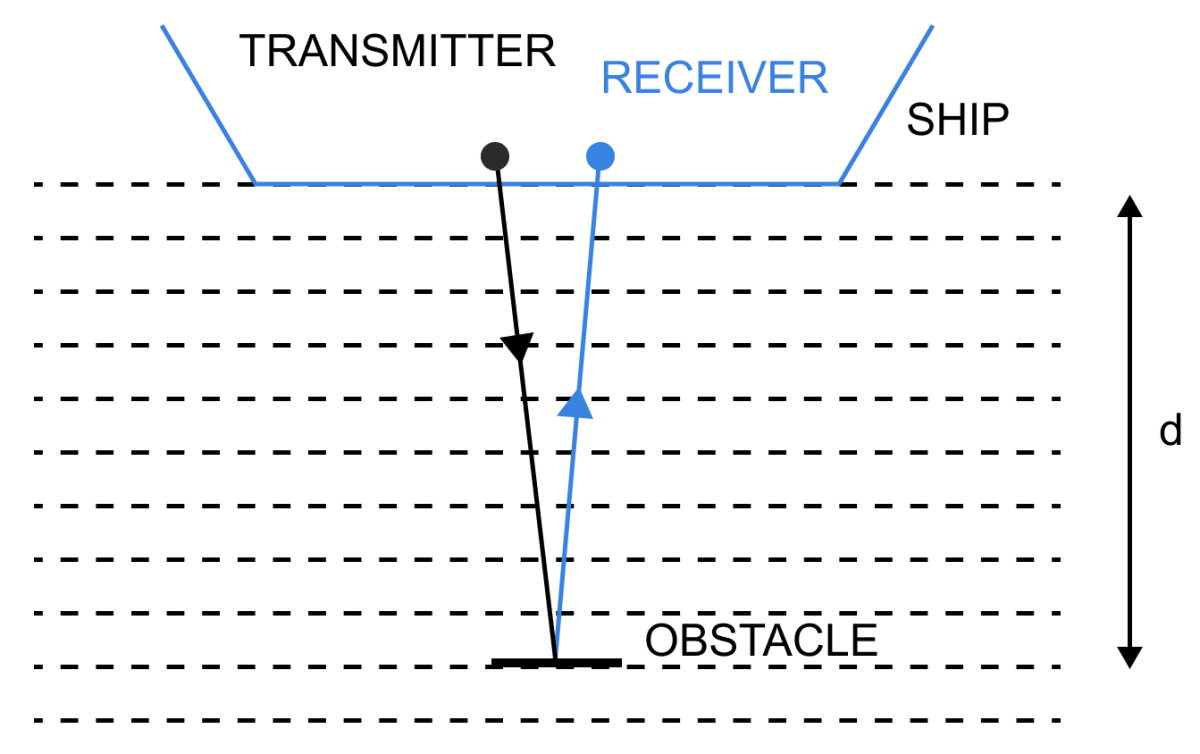To find the distance of obstacle from the ship the time interval (t) between the instant when waves are sent and the instant when waves are received, after reflection from the obstacle is measured.

The distance d of the obstacle from the source is then —

$\text{d} = \dfrac{Vt}{2} \\[0.5em]$

where, V is the speed of ultrasonic wave in water.

The depth of sea can also be found by this method. The process is then called echo depth sounding.

#### Question 16

State the use of echo in medical field.

In medical field, echo method of ultrasonic wave is used for imaging the human organs (such as liver, gall bladder, uterus, womb, etc.) This is called ultrasonography.

Similarly, to obtain the image of human heart echo cardiography is used.

## Multiple Choice Type

#### Question 1

The minimum distance between the source and the reflector in air, to hear an echo, is approximately —

1. 10 m
2. 17 m ✓
3. 34 m
4. 50 m.

To hear the echo distinctly, the minimum distance between the source of sound (or observer) and the reflector in air must be 17 m because if the distance is less than 17 m, the reflected sound will reach the ears before the original sound dies out (i.e., before 0.1 s) and so no echo will be heard.

#### Question 2

To detect the obstacles in their path, bats produce —

1. Infrasonic waves
2. Ultrasonic waves ✓
3. Electromagnetic waves

Bats can produce and detect the sound waves of very high frequency up to about 100 kHz. The audible sound range is from 20 Hz to 20 kHz. Any frequency above 20 kHz is called ultrasonic waves. Hence, we can say that bats produce ultrasonic waves.

## Numericals

#### Question 1

The wavelength of waves produced on the surface of water is 20 cm. If the wave velocity is 24 ms-1, calculate (i) the number of waves produced in one second and (ii) the time in which one wave is produced.

(i) As we know,

Velocity (V) = frequency (f) x wavelength (λ)

Given,

V = 24 ms-1

λ = 20 cm

Converting cm to m, we get,

100 cm = 1 m,

1 cm = $\dfrac{1}{100}$ m = 0.01 m,

Therefore, 20 cm = (20 x 0.01) m = 0.2 m

Hence, λ = 0.2 m

Substituting the values in the formula above, we get,

$24 = f \times 0.2 \\[0.5em] \Rightarrow f = \dfrac{24}{0.2} \\[0.5em] \Rightarrow f = \dfrac{240}{2} \\[0.5em] \Rightarrow f = 120 s ^{-1}\\[0.5em]$

Therefore, the number of waves produced in one second = 120 s-1

(ii) As we know,

Time in which one wave is produced (t) = $\dfrac{1}{f}$

Substituting the values in the formula above, we get,

$t = \dfrac{1}{120} \\[0.5em] \Rightarrow t = 0.0083 \\[0.5em] \Rightarrow t = 0.0083 s \\[0.5em]$

Hence,

Time in which one wave is produced = 8.3 x 10-3 s

#### Question 2

Calculate the minimum distance in air required between the source of sound and the obstacle to hear an echo. Take speed of sound in air = 350 ms-1

As we know,

$\text {Speed of sound (V)} = \dfrac{\text{Total distance travelled (2d)}}{\text {time interval (t)}} \\[0.5em]$

Total distance travelled by the sound in going and then coming back = 2d

Given,

V = 350 ms-1

An important condition for hearing an echo is that the reflected sound should reach the ear only after a lapse of at least 0.1 s after the original sound dies off.

Hence, t = 0.1 s

Substituting the values in the formula above, we get,

$350 = \dfrac{2d}{0.1} \\[0.5em] \Rightarrow 2d = 350 \times 0.1 \\[0.5em] \Rightarrow d = 17.5 m \\[0.5em]$

Hence, the minimum distance in air required between the source of sound and the obstacle to hear an echo = 17.5 m

#### Question 3

What should be the minimum distance between the source and reflector in water so that the echo is heard distinctly? (The speed of sound in water = 1400 ms-1).

As we know,

$\text {Speed of sound (V)} = \dfrac{\text{Total distance travelled (2d)}}{\text {time interval (t)}} \\[0.5em]$

Total distance travelled by the sound in going and then coming back = 2d

Given,

V = 1400 ms-1

As important condition for hearing an echo is that the reflected sound should reach the ear only after a lapse of at least 0.1 s after the original sound dies off.

Hence, t = 0.1 s

Substituting the values in the formula above, we get,

$1400 = \dfrac{2d}{0.1} \\[0.5em] \Rightarrow 2d = 1400 \times 0.1 \\[0.5em] \Rightarrow d = \dfrac{140}{2} \\[0.5em] \Rightarrow d = 70 m \\[0.5em]$

Hence, the minimum distance between the source and reflector in water so that echo is heard distinctly = 70 m

#### Question 4

A man standing 25 m away from a wall produces a sound and receives the reflected sound. (a) Calculate the time after which he receives the reflected sound if the speed of sound in air is 350 ms-1. (b) Will the man be able to hear a distinct echo? Explain the answer.

(a) As we know,

$\text {Speed of sound (V)} = \dfrac{\text{Total distance travelled (2d)}}{\text {time interval (t)}} \\[0.5em]$

Total distance travelled by the sound in going and then coming back = 2d

Given,

d = 25 m

V = 350 ms-1

Substituting the values in the formula above, we get,

$350 = \dfrac{2 \times 25}{t} \\[0.5em] \Rightarrow t = \dfrac{50}{350} \\[0.5em] \Rightarrow t = \dfrac{1}{7} \\[0.5em] \Rightarrow t = 0.143 s \\[0.5em]$

Hence, the time after which he receives the reflected sound = 0.143 seconds

(b) Yes, the man will be able to hear a distinct echo.
As an important condition for hearing an echo is that the reflected sound should reach the ear only after a lapse of at least 0.1 s because original sound persists only for 0.1 s. Hence, a distinct echo is heard because the man receives the reflected sound after 0.143 seconds.

#### Question 5

A RADAR sends a signal to an aeroplane at a distance 300 km away, with a speed of 3 x 108 ms-1. After how much time is the signal received back after reflecting from the aeroplane?

(a) As we know,

$\text {Speed of sound (V)} = \dfrac{\text{Total distance travelled (2d)}}{\text {time interval (t)}} \\[0.5em]$

Total distance travelled by the sound in going and then coming back = 2d

Given,

d = 300 km

Converting km to m, we get,

1 km = 1000 m

Therefore, 300 km = 3,00,000 m

Hence, d = 3 x 105 m

V = 3 x 108 ms-1

Substituting the values in the formula above, we get,

$3 \times 10^8 = \dfrac{2 \times (3 \times 10^5) }{t} \\[0.5em] t = \dfrac{2 \times (3 \times 10^5)}{3 \times 10^8} \\[0.5em] t = 2 \times 10^{-3} \\[0.5em]$

Hence, the signal is received back after 2 x 10-3 s

#### Question 6

A man standing 48 m away from a wall fires a gun. Calculate the time after which an echo is heard. (The speed of sound in air is 320 ms-1).

As we know,

$\text {Speed of sound (V)} = \dfrac{\text{Total distance travelled (2d)}}{\text {time interval (t)}} \\[0.5em]$

Total distance travelled by the sound in going and then coming back = 2d

Given,

d = 48 m

V = 320 ms-1

Substituting the values in the formula above, we get,

$320 = \dfrac{2 \times 48}{t} \\[0.5em] t = \dfrac{2 \times 48}{320} \\[0.5em] t = 0.30 s \\[0.5em]$

Hence, time after which echo is heard = 0.30 s.

#### Question 7

A ship on the surface of water sends a signal and receives it back from the submarine inside water after 4 s. Calculate the distance of the submarine from the ship. (The speed of sound in water is 1450 ms-1).

As we know,

$\text {Speed of sound (V)} = \dfrac{\text{Total distance travelled (2d)}}{\text {time interval (t)}} \\[0.5em]$

Total distance travelled by the sound in going and then coming back = 2d

Given,

t = 4 s

V = 1450 ms-1

Substituting the values in the formula above, we get,

$1450 = \dfrac{2d}{4} \\[0.5em] \Rightarrow d = \dfrac{1450 \times 4}{2} \\[0.5em] \Rightarrow d = 1450 \times 2 \\[0.5em] \Rightarrow d = 2900 m$

Converting m to km

1000 m = 1 km

Therefore, 2900 m = $\dfrac{2900}{1000}$ km = 2.9 km

Hence, distance of the submarine from the ship = 2.9 km

#### Question 8

A pendulum has a frequency of 5 vibrations per second. An observer starts the pendulum and fires a gun simultaneously. He hears an echo from the cliff after 8 vibrations of the pendulum. If the velocity of sound in air is 340 ms-1, find the distance between the cliff and the observer.

As we know,

$\text {Speed of sound (V)} = \dfrac{\text{Total distance travelled (2d)}}{\text {time interval (t)}} \\[0.5em]$

5 vibrations are produced in 1 second.

Therefore, time for 8 vibrations = ($\dfrac{1}{5}$ x 8) s= $\dfrac{8}{5}$ s = 1.6 s

Hence, t = 1.6 s

V = 340 ms-1

Substituting the values in the formula above, we get,

$340 = \dfrac{2d}{1.6} \\[0.5em] \Rightarrow d = \dfrac{340 \times 1.6}{2} \\[0.5em] \Rightarrow d = 340 \times 0.8 \\[0.5em] \Rightarrow d = 272 m$

Therefore, the distance between the cliff and the observer = 272 m

#### Question 9

A person standing between two vertical cliffs produces a sound. Two successive echoes are heard at 4s and 6s. Calculate the distance between the cliffs.

(Speed of sound in air = 320 ms-1)

[HINT — First echo will be heard from the nearer cliff and the second echo from the farther cliff.]

As we know,

$\text {Speed of sound (V)} = \dfrac{\text{Total distance travelled (2d)}}{\text {time interval (t)}} \\[0.5em]$

Total distance travelled by the sound in going and then coming back = 2d

Given,

t1 = 4 s

t2 = 6 s

V = 320 ms-1 and

First echo will be heard from the nearer cliff and the second echo from the farther cliff.

Hence, Distance between cliffs = d1 + d2

Therefore, substituting the values in the formula above, we get,

For t1

$320 = \dfrac{2d_1 }{4} \\[0.5em] \Rightarrow d_1 = \dfrac{320 \times 4}{2} \\[0.5em] \Rightarrow d_1 = 640 m$

So, For t2

$320 = \dfrac{2d_2}{6} \\[0.5em] \Rightarrow d_2 = \dfrac{320 \times 6}{2} \\[0.5em] \Rightarrow d_2 = 960 m$

Distance between cliffs = d1 + d2

= 640 + 960

= 1600 m

Hence, distance between cliffs = 1600 m

#### Question 10

A person standing at a distance x in front of a cliff fires a gun. Another person B standing behind the person A at a distance y from the cliff hears two sounds of the fired shot after 2 s and 3 s respectively. Calculate x and y (take speed of sound 320 ms-1)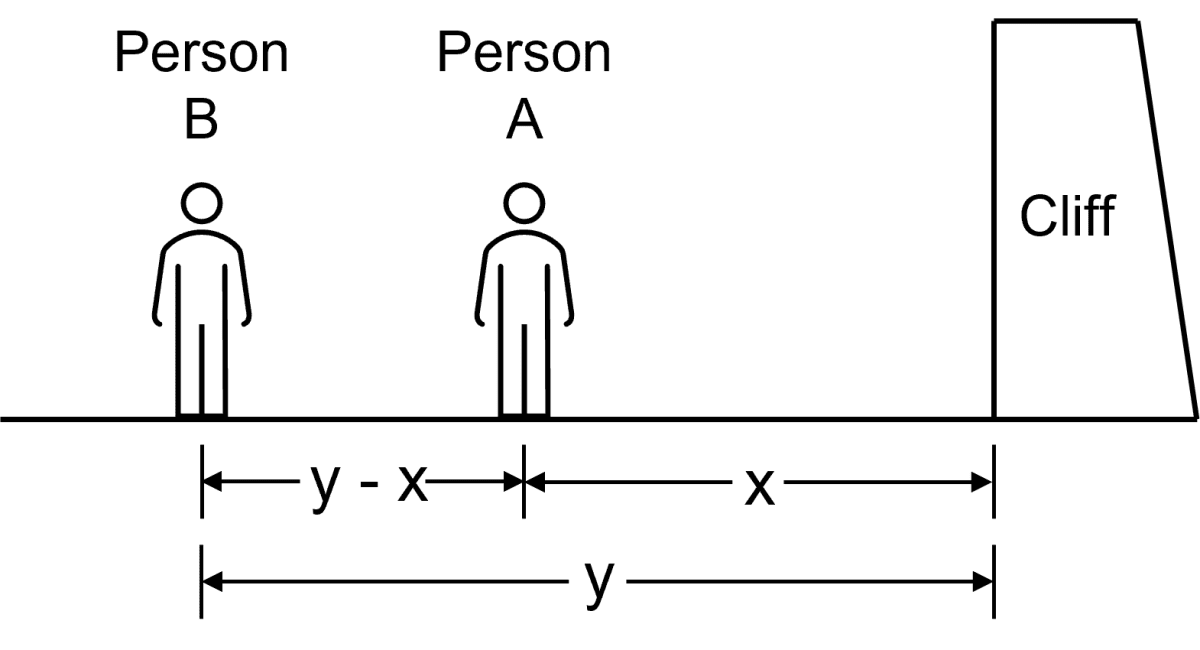Given,

speed of sound 320 ms-1

As we know,

$\text {Speed} = \dfrac{\text {distance}}{\text{time}}$

The person B hears two sounds of the fired shot, the first one is direct from the gun while another sound comes after reflection from the cliff

In the case of first sound for person B, when t = 2s (i.e., when the gun was fired)

Substituting the values in the formula above, we get,

$320 = \dfrac{\text {y - x}}{\text{2}} \\[0.5em] \text {y - x} = 640 \\[0.5em]$

Hence, y - x = 640 m     [Equation 1]

In the case of second sound for person B, when t = 3s (i.e. after reflection from the cliff)

Substituting the values in the formula above, we get,

$320 = \dfrac{\text {y + x}}{\text{3}} \\[0.5em] \text {y + x} = 960 \\[0.5em]$

Hence, y + x = 960 m     [Equation 2]

Adding equation (1) and (2), we get,

y - x = 640     [Equation 1]

y + x = 960     [Equation 2]

$2y = 1600 \\[0.5em] y = \dfrac{1600}{2} \\[0.5em] \Rightarrow y = 800 m \\[0.5em]$

Therefore, y = 800 m

Substituting the value of y in eqn 1 we get the value of x,

$800 - x = 640 \\[0.5em] \Rightarrow x = 800 - 640 \\[0.5em] \Rightarrow x = 160 \\[0.5em]$

Therefore, x = 160 m

Hence,

x = 160 m and y = 800 m

#### Question 11

On sending an ultrasonic wave from a ship towards the bottom of a sea, the time interval between sending the wave and receiving it back is found to be 1.5 s. If the velocity of the wave in sea water is 1400 ms-1, find the depth of sea.

As we know,

$\text {Speed of sound (V)} = \dfrac{\text{Total distance travelled (2d)}}{\text {time interval (t)}} \\[0.5em]$

Total distance travelled by the sound in going and then coming back = 2d

Given,

t = 1.5 s

V = 1400 ms-1

Substituting the values in the formula above, we get,

$1400 = \dfrac{2d}{1.5} \\[0.5em] \Rightarrow d = \dfrac{1400 \times 1.5}{2} \\[0.5em] \Rightarrow d = 700 \times 1.5 \\[0.5em] \Rightarrow d = 1050 m$

Therefore, the depth of the sea = 1050 m

#### Question 12

Figure below shows the distance-displacement graph of two waves A and B. Compare (i) the amplitude, (ii) the wavelength of the two waves.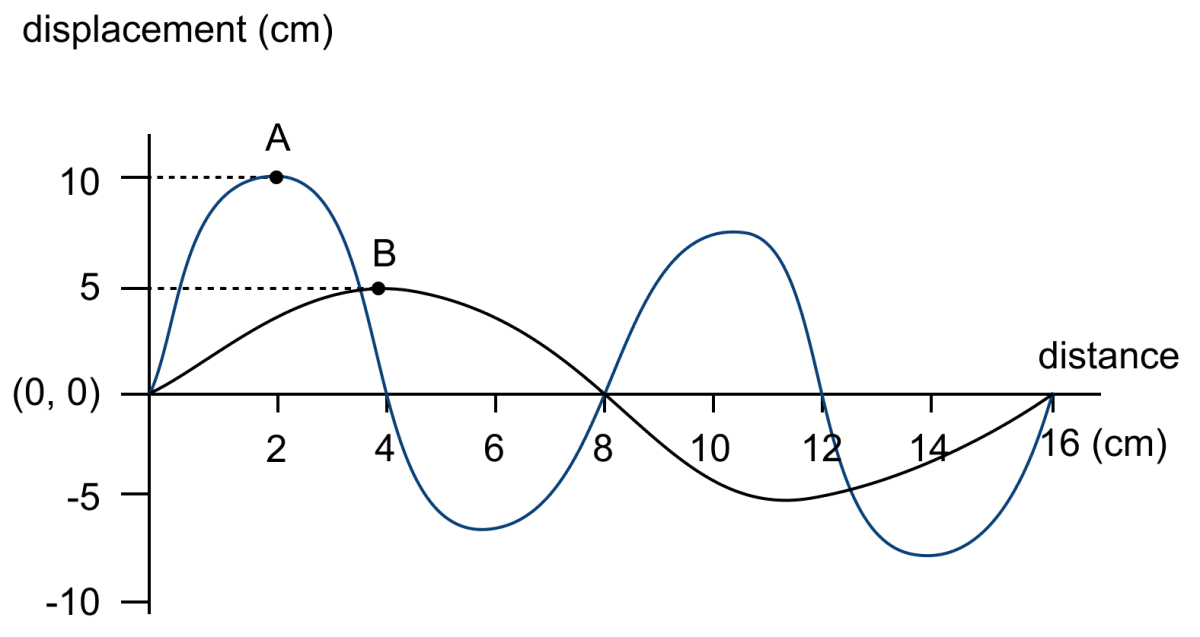(i) Amplitude = maximum displacement from the mean position.

From graph,

For A, Amplitude = 10 cm and

For B, Amplitude = 5 cm

Ratio between the two amplitudes —

$\dfrac{10}{5}$ = $\dfrac{2}{1}$ = 2 : 1

Therefore, the ratio of the two amplitudes = 2 : 1

(ii) For A, Wavelength λ1 = 8 cm

For B, Wavelength λ2 = 16 cm

Ratio between the two Wavelengths —

$\dfrac{8}{16}$ = $\dfrac{1}{2}$ = 1 : 2

Therefore, the ratio of the two wavelengths = 1 : 2

## Exercise 7(B)

#### Question 1

What do you understand by the natural vibrations of a body? Give one example.

The periodic vibrations of a body in the absence of any external force on it, are called the natural (or free) vibrations.

For example —

A body clamped at one point when disturbed slightly from its rest position, starts vibrating. The vibrations so produced are called the natural or free vibrations of the body.

#### Question 2

What is meant by the natural frequency of vibrations of a body? Name one factor on which it depends?

The natural frequency is the frequency at which a body tends to oscillate in the absence of any driving or damping force.

The natural frequency of vibrations of a body depends on the shape and size (or structure) of the body.

#### Question 3

(a) Draw a graph between displacement and time for a body executing natural vibrations

(b) Where can a body execute the natural vibrations?

(a) Below is the graph between displacement and time for a body executing natural vibrations: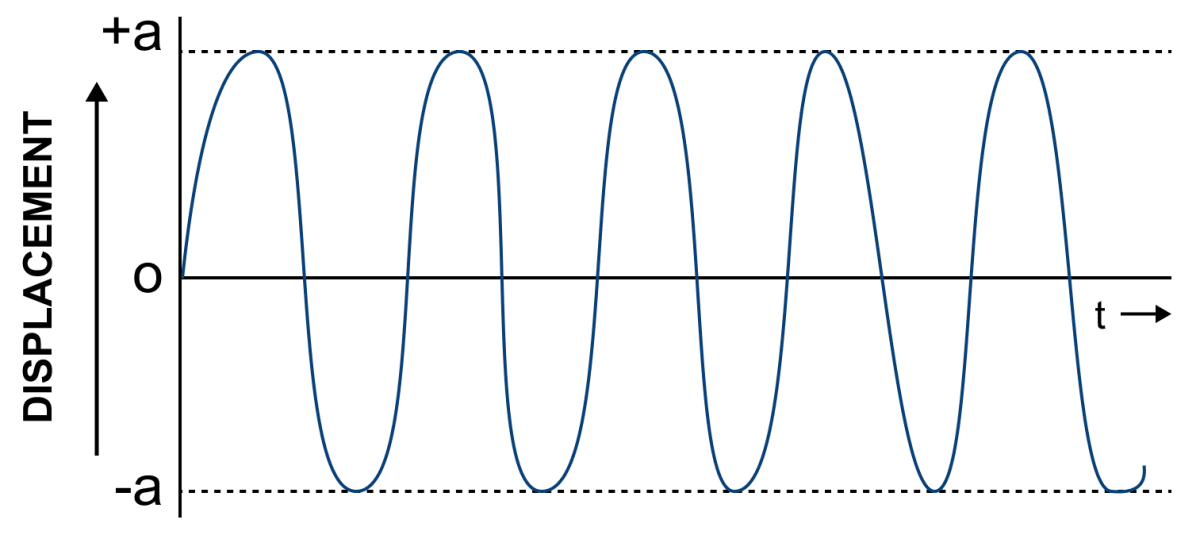(b) The natural vibrations can occur only in vacuum.

However, in practice it is very difficult to have vacuum. Hence, it is very difficult to realise such vibrations in real life.

#### Question 4

State one condition for a body to execute the natural vibrations.

The natural vibrations of a body actually occur only in vacuum because the presence of medium around the body offers some resistance due to which the amplitude of vibration does not remain constant, but it continuously decreases.

#### Question 5

(a) Name one factor on which the frequency of sound emitted due to vibration in an air column depends.

(b) How does the frequency depend on the factor stated in part (a)?

(a) The frequency of sound emitted due to vibration in an air column depends on the length of air column.

(b) As we know,

Frequency (f) = $\dfrac{1}{l}$

Hence, frequency is inversely proportional to length, so in order to increase the frequency, the length has to be decreased.

#### Question 6

State one way of increasing the frequency of a note produced by an air column.

As we know,

Frequency (f) = $\dfrac{1}{l}$

Hence, frequency is inversely proportional to length.

Therefore, an increase in the frequency of the note can be attained, by decreasing the length of the air column.

#### Question 7

State two ways of increasing the frequency of vibrations of a stretched string.

As we know,

frequency (f) = $\dfrac{1}{2l} \sqrt\dfrac{T}{πr^2d}$

Hence, the frequency of vibrations of a stretched string can be increased —

(i) by increasing the tension in the string

(ii) by decreasing the length of the string.

#### Question 8

How does the frequency of sound given by a stretched string depend on its (a) length, (b) tension?

(a) As we know,

frequency (f) = $\dfrac{1}{2l} \sqrt\dfrac{T}{πr^2d}$

Hence, the frequency of sound given by a stretched string is inversely proportional to the length of the string.

f ∝ $\dfrac{1}{l}$

(b) The frequency of sound given by a stretched string is directly proportional to the square root of the tension in the string.

f ∝ $\sqrt T$

#### Question 9

What adjustments would you make for tuning a stringed instrument for it to emit a note of a desired frequency?

As we know,

frequency (f) = $\dfrac{1}{2l} \sqrt\dfrac{T}{πr^2d}$

Hence, frequency of a desired note can be obtained by altering the following —

(a) Length of the string — In order to increase the frequency, the length of the string should be decreased.

(b) Radius (thickness) of the string — In order to increase the frequency, the radius of the string should be decreased.

(c) Tension in the string — In order to increase the frequency, the tension in the string should be increased.

#### Question 10

The diagram given below shows three ways in which the string of length l in an instrument can vibrate.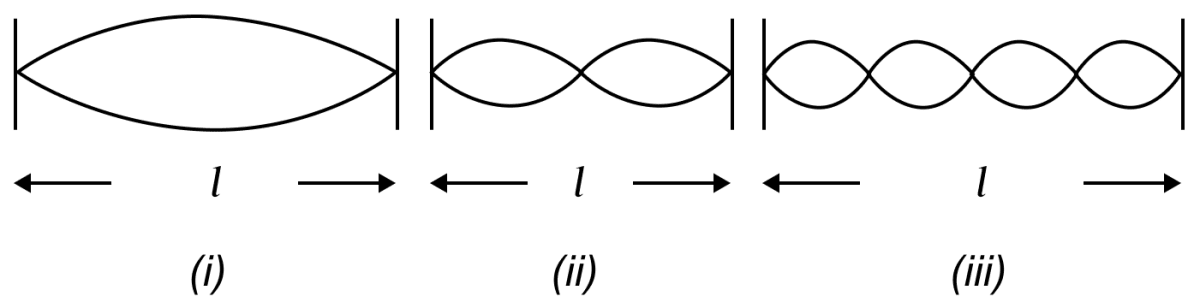(a) Which of the diagram shows the principal note ?

(b) Which vibration has the frequency four times that of the first ?

(c) Which vibration is of longest wavelength ?

(d) What is the ratio of the frequency of vibrations in diagrams (i) and (ii) ?

(a) The principal note is shown in part (i) of the diagram.

(b) Part (iii) of the diagram has frequency four times that of the first.

(c) The vibration in part (i) has the longest wavelength.

(d) Let the frequency of part (i) be f. Then frequency of part (ii) is 2f.

Hence, the ratio between (i) and (ii) is —

$\dfrac{f}{2f} = \dfrac{1}{2}$

Therefore, the ratio between the frequency of part (i) and part (ii) is 1 : 2

#### Question 11

Explain why strings of different thickness are provided on stringed instrument.

[Hint — Natural frequency of vibration of a stretched string is inversely proportional to the radius (or thickness) of string so notes of different frequencies can be obtained by producing vibrations in the different strings]

As we know,

frequency (f) = $\dfrac{1}{2l} \sqrt\dfrac{T}{πr^2d}$

So, we can say that the natural frequency of vibration of a stretched string is inversely proportional to the radius of the string.

Hence, in order to produce sound waves of different frequencies, strings of different thickness are provided on a stringed instrument.

#### Question 12

A blade, fixed at one end, is made to vibrate by pressing its other end and then releasing it. State one way in which the frequency of vibrations of the blade can be lowered.

The frequency of vibrations of the blade can be lowered by increasing the length of the blade or by sticking a small weight on the blade at its free end, so as to increase its mass.

#### Question 13

How does the medium affect the amplitude of the natural vibrations of a body?

When a body is made to vibrate in a medium, the amplitude of the vibrating body continuously decreases with time because energy is lost in doing work against the force of friction offered by the medium and ultimately the body stops vibrating.

Such vibrations are called the damped vibrations.

#### Question 14

What are the damped vibrations? How do they differ from free vibrations? Give one example of each.

The periodic vibrations of a body of decreasing amplitude in presence of a resistive force are called the damped vibration.

In the case of damped vibrations, the amplitude of vibrations gradually decreases with time and ultimately it ceases whereas in the case of free vibrations the amplitude of vibrations remains constant and it continues forever.

Example of damped vibration — A tuning fork when stroked on a rubber pad, executes the damped vibrations in air.

Example of free vibration — The vibrations of a constant amplitude can only occur in vacuum. Since, in practice, it is very difficult to have vacuum. Hence, it is very difficult to realise such vibrations in practice.

#### Question 15

The diagram in figure below shows the displacement-time graph of a vibrating body.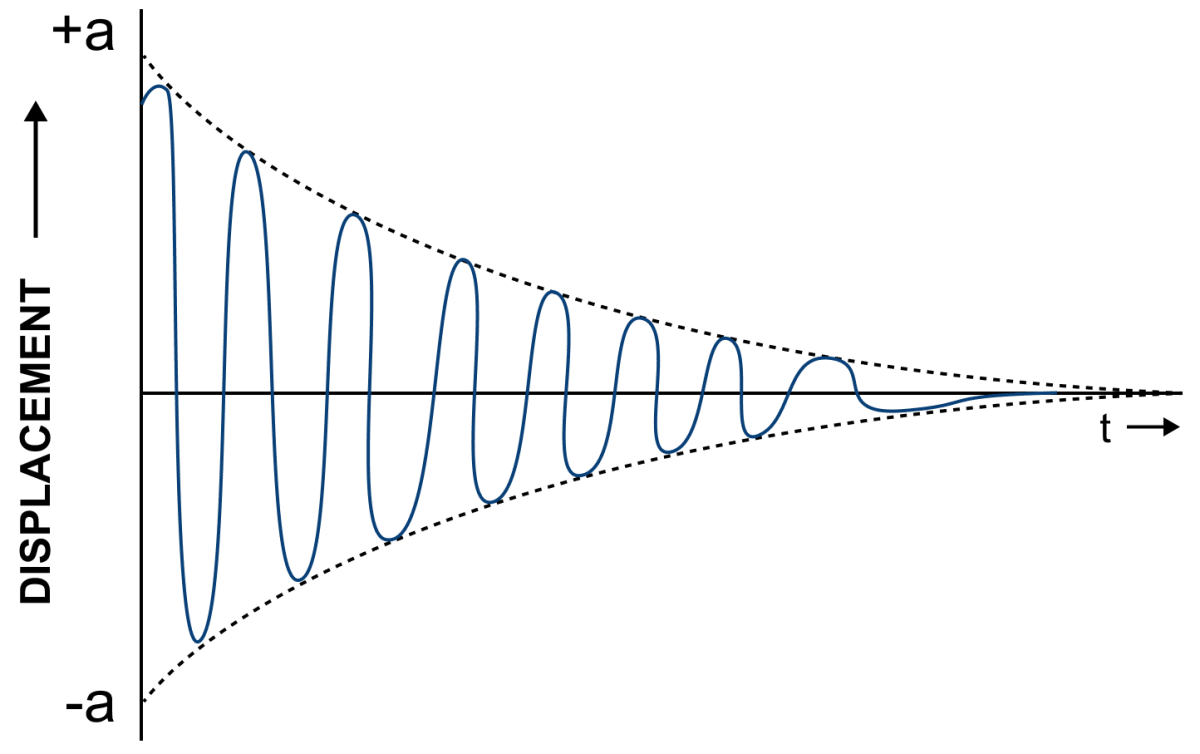(i) Name the kind of vibrations.

(ii) Give one example of such vibration.

(iii) Why is the amplitude of vibrations gradually decreasing?

(iv) What happens to the vibrations of the body after some time?

(i) The above shown displacement-time graph of a vibrating body represents damped vibrations.

(ii) Example of damped vibration — A tuning fork when stroked on a rubber pad, executes the damped vibrations in air.

(iii) The amplitude of vibrations decreases due to the frictional force.

The frictional force at any instant is proportional to the velocity of the body and it has the tendency to resist the motion.

As a result, the energy of the vibrating body continuously dissipates in doing work against the force of friction and so its amplitude gradually decreases.

(iv) After some time, when the body has lost all its energy, it stops vibrating.

#### Question 16

A tuning fork is vibrating in air. State whether the vibrations are natural or damped.

A tuning fork when stroked on a rubber pad, executes damped vibrations in air.

#### Question 17

Draw a sketch showing the displacement against time for a body executing the damped vibrations.

The displacement of a body executing damped vibrations, against time is shown below.#### Question 18

What are the forced vibrations? Give one example to illustrate your answer.

The vibrations of a body which take place under the influence of an external periodic force acting on it, are called forced vibrations.

Example — The vibrations produced in the diaphragm of a microphone sound box with frequencies corresponding to the speech of the speaker, are the forced vibrations.

#### Question 19

On keeping the stem of a vibrating tuning fork on the surface of a table, a loud sound is heard. Give reason.

When the stem of a vibrating tuning fork is pressed against the top of a table, the forced vibrations are produced on the surface of table.

The table top has a much larger vibrating area than the tuning fork, so the forced vibrations of the table top send forth a greater energy and hence, produce a larger sound ( or more intense ) than that produced by the fork.

#### Question 20

State two differences between natural and forced vibrations.

Differences between the natural and forced vibrations are as follows —

Natural VibrationsForced Vibrations
The vibrations of a body in absence of any resistive or external force are called natural vibrations.The vibrations of a body in a medium in presence of an external periodic force are called forced vibrations.
The frequency of vibrations remains constant.The frequency of vibration changes with change in the frequency of the applied force.

#### Question 21

What is meant by resonance? Describe a simple experiment to illustrate the phenomenon of resonance and explain it.

Resonance is a special case of forced vibrations.

When the frequency of the externally applied periodic force on a body is equal to its natural frequency, the body readily begins to vibrate with an increased amplitude. This phenomenon is known as resonance.

Experiment — Mount two identical tuning forks A and B, of the same frequency on two separate sound boxes such that their open ends face each other as shown in figure below.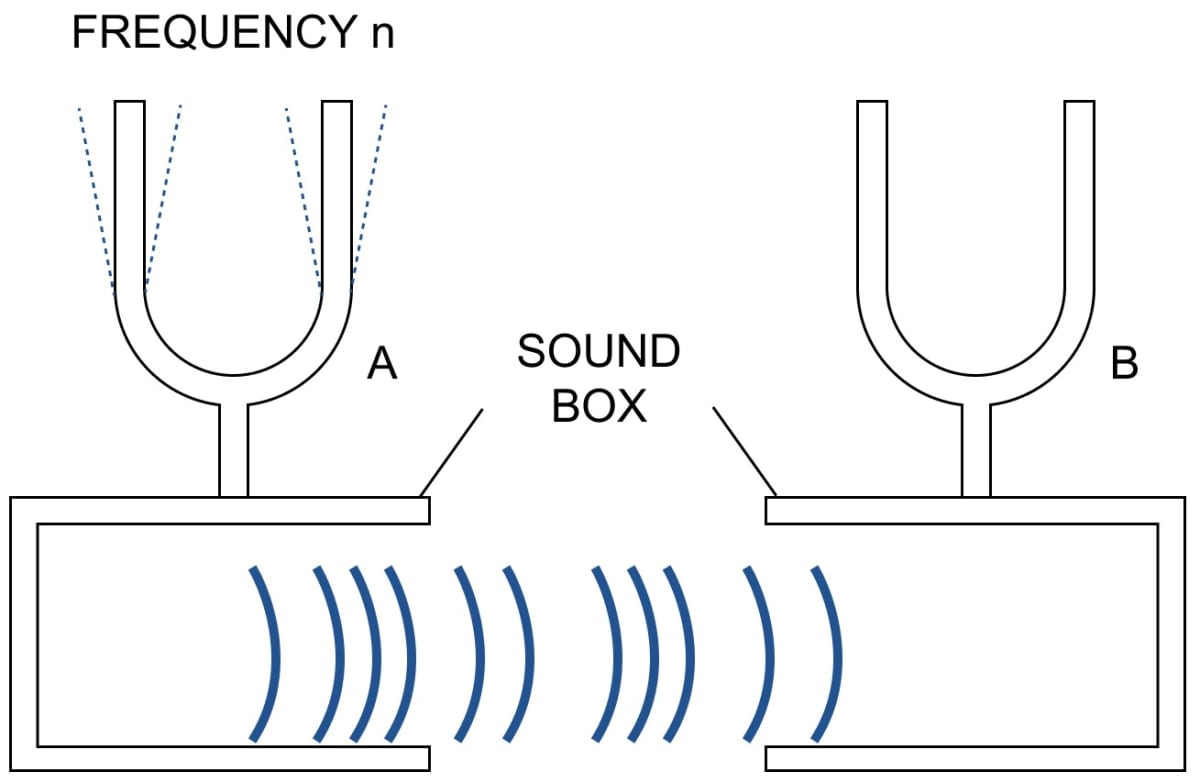When the prong of one of the tuning forks say, A is struck on a rubber pad, it starts vibrating. On putting the tunning fork A on its sound box, we find that the other tuning fork B also starts vibrating and a loud sound is heard.

The vibrating tuning fork A produces the forced vibration in the air column of its sound box. These vibrations are of large amplitude because of large surface area of air in the sound box.

These vibrations are communicated to the sound of the fork B. The air column of B starts vibrating with the frequency of fork A. Since the frequency of these vibrations is same as the natural frequency of the fork B, the fork B picks up these vibrations under resonance.

#### Question 22

State the condition for the resonance to occur.

Resonance occurs only when the applied force causes forced vibration in the body and the frequency of the applied force is exactly equal to the natural frequency of the vibrating body.

#### Question 23

Complete the following sentence:

Resonance is a special case of ______ vibrations, when frequency of the driving force is _______ natural frequency of the driven body.

Resonance is a special case of forced vibrations, when the frequency of the driving force is equal to the natural frequency of the driven body.

#### Question 24

State two differences between forced and resonant vibrations

The difference between the forced and resonant vibrations are as follows:

Forced vibrationsResonant vibrations
The vibrations of a body under an external periodic force of frequency different from the natural frequency of the body, are called Forced vibrations.The vibrations of a body under an external periodic force of frequency exactly equal to the natural frequency of the body are called Resonant vibrations.
The amplitude of vibration is small.The amplitude of vibration is very large.

#### Question 25

Why is a loud sound heard at resonance?

At resonance, the body vibrates with a large amplitude thus conveying more energy to the ears, so a loud sound is heard.

#### Question 26

Figure below shows two tuning forks A and B of the same frequency mounted on two separate sound boxes with their open ends facing each other. The fork A is set into vibration. (a) Describe your observation. (b) State the principle illustrated by this experiment.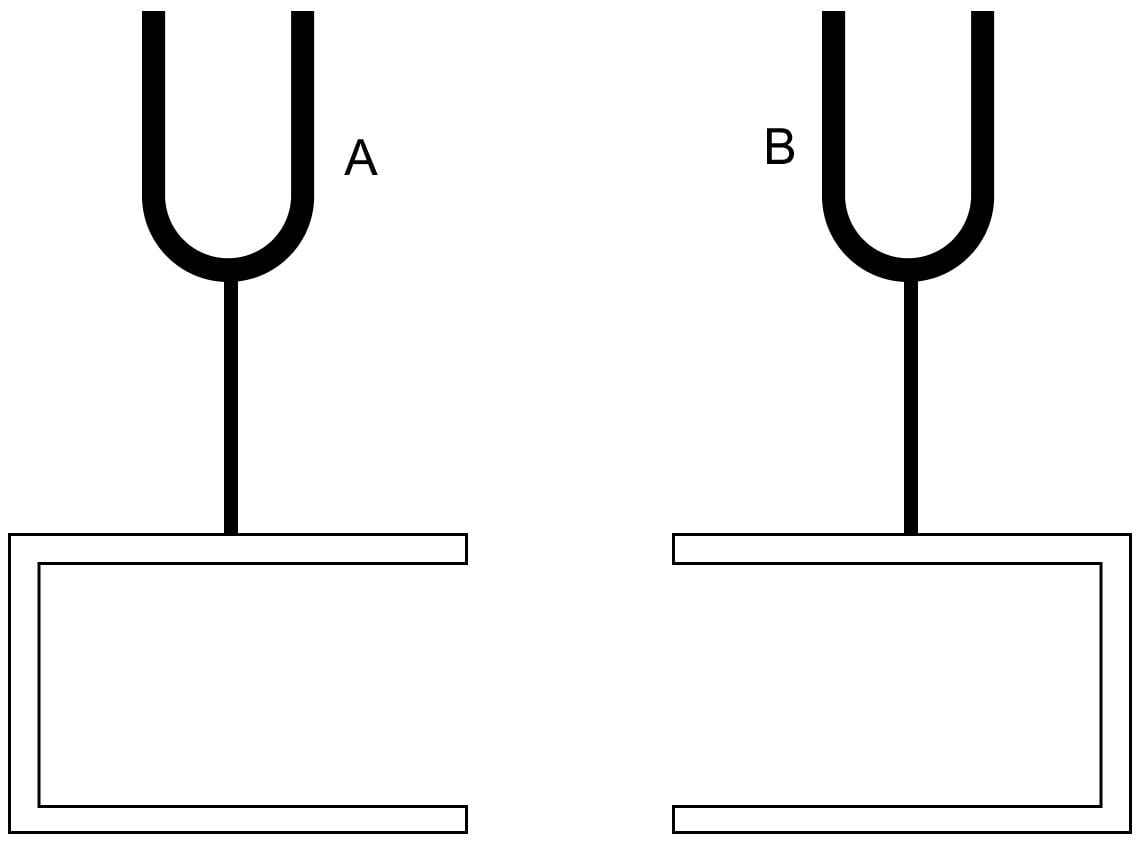(a) During the above experiment, we observe that,

When the prong of one of the tuning forks say, A is struck on a rubber pad, it starts vibrating. On putting the tunning fork A on its sound box, we find that the other tuning fork B also starts vibrating and a loud sound is heard.

The vibrating tuning fork A produces the forced vibration in the air column of its sound box. These vibrations are of large amplitude because of large surface area of air in the sound box.

These vibrations are communicated to the sound box of the fork B. The air column of B starts vibrating with the frequency of fork A. Since the frequency of these vibrations is same as the natural frequency of the fork B, the fork B picks up these vibrations under resonance.

(b) The principle illustrated by the above experiment is Resonance.

#### Question 27

In the figure shown below A, B, C and D are the four pendulums suspended from the same elastic string XY. The lengths of pendulum A and D are equal, while the length of pendulum B is shorter and of the pendulum C is longer. Pendulum A is set into vibrations.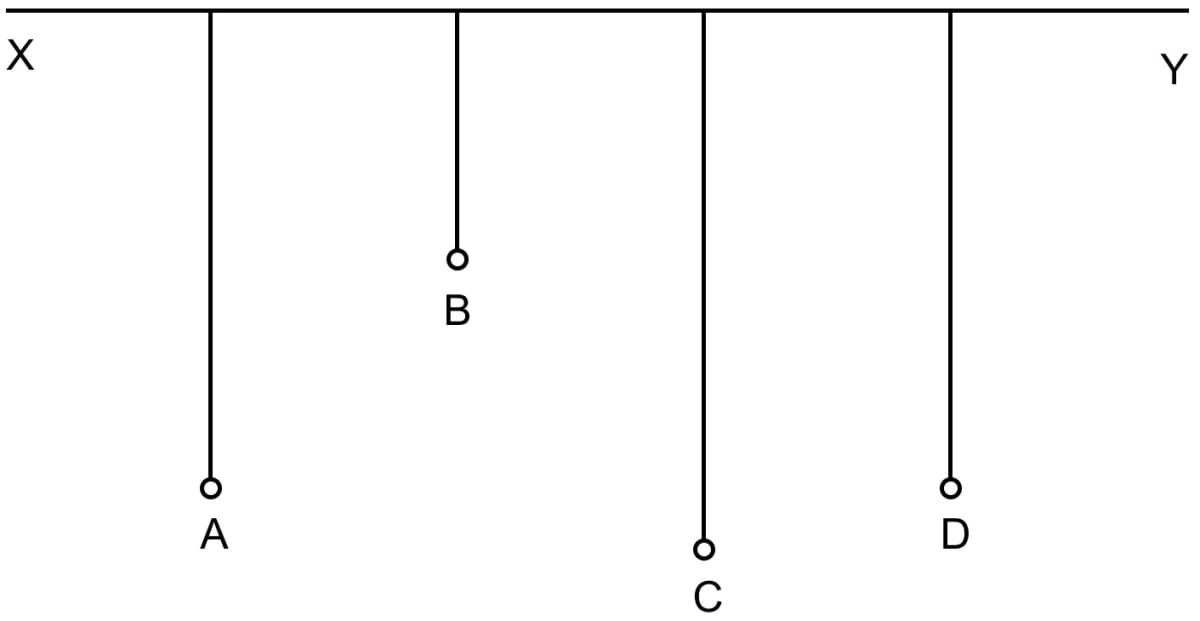(a) What is your observation about the vibrations of pendulum D?

(b) Give reason for your observation in part (a).

(c) What type of vibrations take place in pendulums B and C?

(d) Give reason for the answer in part (c)

(a) When pendulum A is set into vibrations, pendulum D also starts vibrating initially with a small amplitude and ultimately it acquires the amplitude same as pendulum A initially had.

When the amplitude of pendulum D becomes maximum, the amplitude of pendulum A becomes minimum because of the sharing energy by them.

Thereafter, the amplitude of pendulum B decreases and that of A increases. This process continues.

(b) Pendulum D is in state of resonance with pendulum A because the natural frequency of pendulum D is equal to that of A (as both have same length), and therefore there is an exchange of energy between the pendulums A and D.

When the amplitude of pendulum D increases, the amplitude of pendulum A decreases and vice versa. The pendulum D, therefore, vibrates with the frequency of pendulum A and it remains in phase with pendulum A.

(c) The vibrations of both pendulums B and C are of a very small amplitude.

(d) The length of pendulums B and C is not equal to the length of pendulum A, so they vibrate with small amplitude because they are under forced vibrations and not resonance.

#### Question 28

A vibrating tuning fork, held over an air column of a given length with its one end closed, produces a loud audible sound. Name the phenomenon responsible for it and explain the observation.

The phenomenon responsible for producing a loud audible sound when a vibrating tuning fork, held over an air column of a given length with its one end closed is known as resonance.

The frequency of air column becomes equal to the frequency of the tuning fork vibrating over its mouth, a loud sound is heard due to resonance.

#### Question 29

In figure given below, A, B, C and D represent the test tubes each of height 20 cm which are filled with water up to heights of 12 cm, 14 cm, 16 cm and 18 cm respectively. If a vibrating tuning fork is placed over the mouth of test tube D, a loud sound is heard.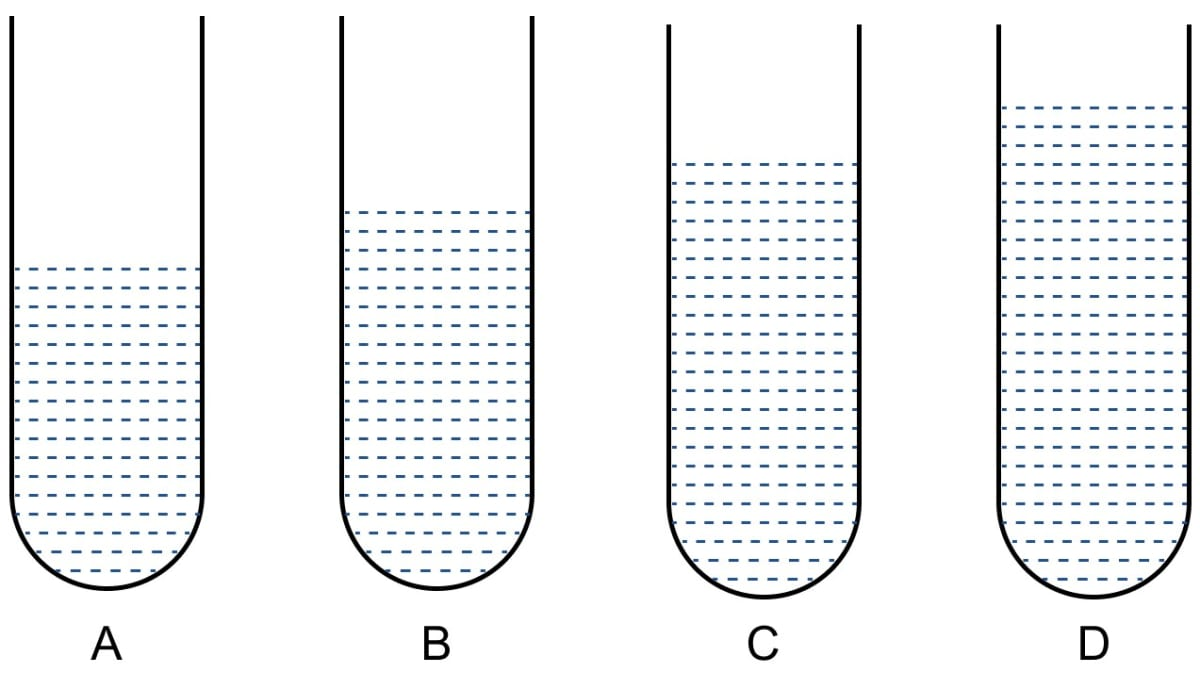(a) Describe the observations with the tubes A, B and C when the vibrating tuning fork is placed over the mouth of these tubes.

(b) Give the reason for your observation in each tube.

(c) State the principle illustrated by the above experiment.

(a) No loud sound is heard with the tubes A and C, but a loud sound is heard with the tube B.

(b) Frequency of air column in tube D is equal to the frequency of tuning fork. Resonance occurs with the air column in tube B whereas no resonance occurs with the air column of tubes A and C. The frequency of vibrations of the air column in tube B is same as the frequency of vibrations of the air column in tube D because the length of the air column in tube D is 20 – 18 = 2 cm and that in tube B is 20 – 14 = 6 cm (i.e., three time). On the other hand, the frequency of vibrations of the air column in tubes A and C is not equal to the frequency vibrations of air column in tube D.

(c) When the frequency of vibrations of the air column is equal to the frequency of the vibrating tuning fork, resonance occurs.

#### Question 30

When a troop crosses a suspension bridge, the soldiers are asked to break their steps. Why?

When a troop crosses a suspension bridge, the soldiers are asked to break their step, because when the soldiers march in steps, each soldier exerts a periodic force in same phase and therefore the bridge executes the forced vibrations of frequency equal to the frequency of their steps.

Now, if the frequency of the steps becomes equal to the natural frequency of the bridge, the bridge will vibrate with large amplitude due to resonance and hence, the suspension bridge may collapse.

#### Question 31

Why are the stringed instruments like guitar provided with a hollow soundbox?

The stringed instruments like guitar are provided with a hollow soundbox containing air because when strings are made to vibrate, forced vibrations are produced in air of the sound box.

The surface area of air in the sound box is large, so the forced vibrations of air send forth a greater energy and cause a loud sound.

#### Question 32

How do you tune your radio set to a particular station? Name the phenomenon involved in doing so and define it.

When we want to tune a radio (or TV) receiver, we merely adjust the values of the electronic components to produce vibrations of frequency equal to that of the radio waves which we want to receive.

When both the frequencies match, resonance occurs and only the energy of signal of that particular frequency is received from the waves present in space, leaving the signals of other frequency of the receiver circuit. The signal received is then amplified in the receiver set.

The phenomenon involved is Resonance.

Resonance is a special case of forced vibrations. When the frequency of an externally applied periodic force on a body is equal to its natural frequency, the body readily begins to vibrate with an increased amplitude.

## Multiple Choice Type

#### Question 1

A wire stretched between two fixed supports, is plucked exactly in the middle and then released. It executes (neglect the resistance of the medium) —

1. resonant vibrations
2. natural vibrations ✓
3. damped vibrations
4. forced vibrations.

When a wire stretched between the two fixed supports, is plucked exactly in the middle and then released, it will experience natural vibrations as no external force is acting on it and the vibrations are under restoring force.

The amplitude of vibrations remains constant and will continue forever as resistance of the medium is negligible.

#### Question 2

When a body vibrates under a periodic force, the vibrations of the body are —

1. natural vibrations
2. damped vibrations
3. forced vibration ✓
4. resonant vibrations.

The vibrations of a body which take place under the influence of an external periodic force acting on it, are called the forced vibrations.

#### Question 3

A tuning fork of frequency 256 Hz will resonate with another tuning fork of frequency —

1. 128 Hz
2. 256 Hz ✓
3. 384 Hz
4. 512 Hz

When the frequency of fork A is same as the natural frequency of fork B, the amplitude of oscillations are very large and the fork B is in resonance with fork A.

Hence, a tuning fork of frequency 256 Hz will resonate only with another tuning fork of frequency 256 Hz.

## Exercise 7(C)

#### Question 1

Name three characteristics of a musical sound.

The three characteristics of a musical sound are —

1. Loudness
2. Pitch (or shrillness)
3. Quality (or timbre)

#### Question 2

(a) Which of the following quantity determines the loudness of a sound wave?

(i) wavelength,

(ii) frequency, and

(iii) amplitude.

(b) How is loudness related to the quantity mentioned in the above part (a)

(a) The loudness of a sound wave is determined by the amplitude of the wave.

If two waves have same frequency and same wave form (sine wave), but they differ in amplitude then the loud sound corresponds to the wave of large amplitude.

(b) Loudness is proportional to the square of the amplitude

Loudness ∝ (amplitude) 2

When a body vibrates with a greater amplitude, it sends forth a greater amount of energy and hence the energy received by the ear drum is large, so the sound appears larger.

#### Question 3

If the amplitude of a wave is doubled, what will be the effect on its loudness?

As we know, that loudness is proportional to the square of the amplitude

Loudness ∝ (amplitude) 2

Therefore, if amplitude = 2a

$\text{L} = (\text{2a})^2 \\[0.5em] \text{L} = \text{4a}^2 \\[0.5em]$

Hence, if amplitude of a wave is doubled loudness will become four times.

#### Question 4

Two waves of the same pitch have amplitudes in the ratio 1 : 3. What will be the ratio of their (i) loudness and (ii) frequencies?

Given,

a1 : a2 = 1 : 3

(i) As we know, that loudness is proportional to the square of the amplitude

Loudness ∝ (amplitude) 2

Therefore,

$\dfrac{L_1}{L_2} = \Big(\dfrac{a_1}{a_2}\Big)^2 \\[0.5em]$

Substituting the values, we get

$\dfrac{L_1}{L_2} = \Big(\dfrac{1}{3}\Big)^2 \\[0.5em] \dfrac{L_1}{L_2} = \dfrac{1}{9}$

Hence, ratio of loudness between the two waves = 1 : 9

(ii) Pitch of a wave depends on its frequency and if two waves have same frequencies then their pitch will be same.

Hence, ratio of pitch between the two waves = 1 : 1.

#### Question 5

How does the wave pattern of a loud note differ from the soft note? Draw a diagram.

Loudness is the characteristic by virtue of which a loud sound can be distinguished from a faint one, both having the same pitch and quality.

The figure below shows how a wave pattern of a loud note differ from the soft note.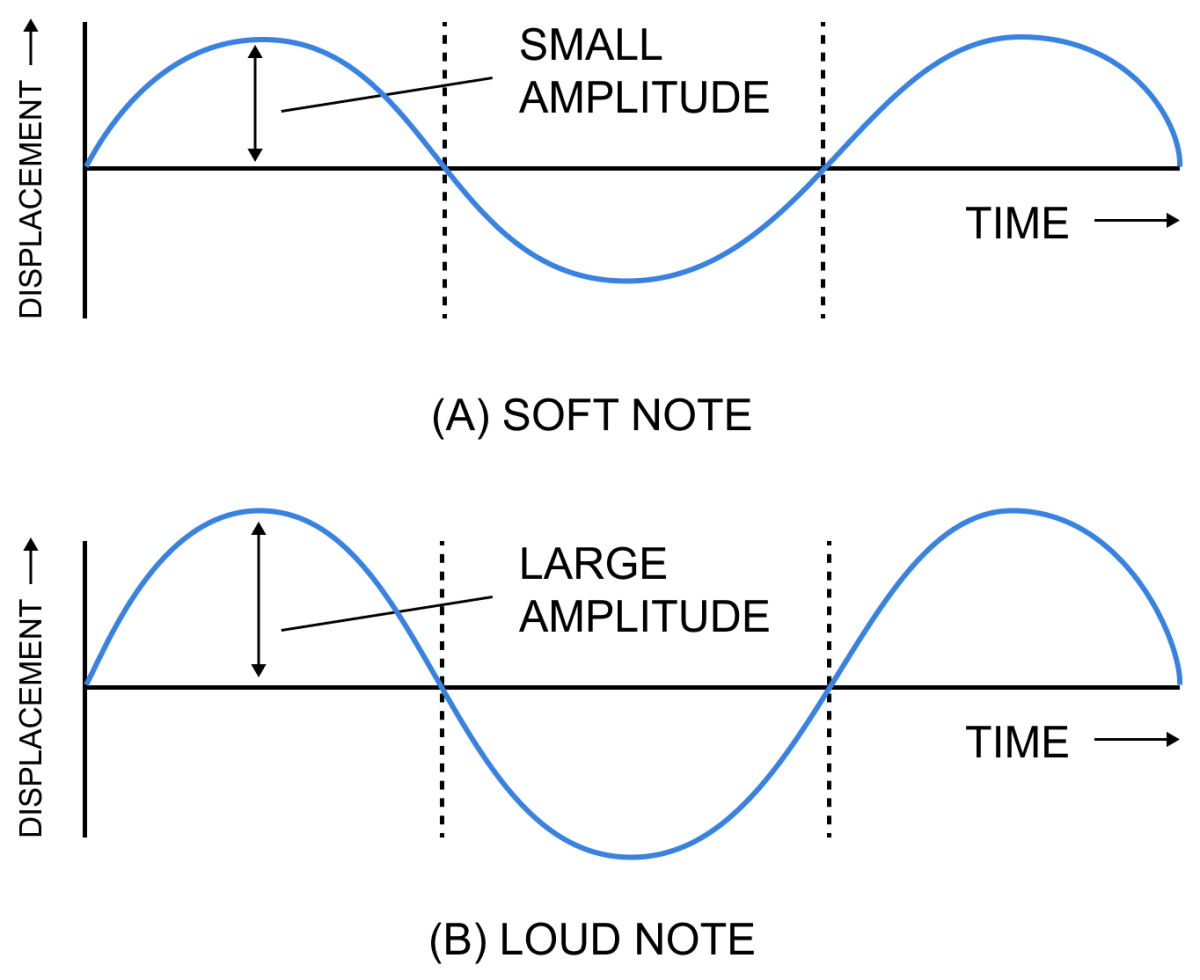#### Question 6

Name the unit in which the loudness of sound is measured?

The unit of measurement of loudness of sound is phon.

#### Question 7

Why is the loudness of the sound heard by a plucked wire increased when it is mounted on a sound board?

As we know, loudness of a sound depends on the surface area of the vibrating body. A large vibrating area sends forth a greater amount of energy. Hence, larger the surface area of the vibrating body, louder is the sound heard.

The surface area of air in the sound board is large, so the forced vibrations of air send forth greater energy and hence, causes a louder sound.

#### Question 8

Define the term intensity of a sound wave. State the unit in which it is measured.

The intensity of the sound wave at a point of the medium is the amount of sound energy passing per second normally through a unit area at that point.

Greater the energy carried by a sound wave, greater is the intensity of sound.

The unit of intensity of the sound wave is watt per metre2 (W m-2).

#### Question 9

How is the loudness of sound related to the intensity of wave producing it?

Relationship between loudness (L) and intensity (I) is given by:

L = K log 10 I

where, K is constant of proportionality.

Hence, loudness increases with the increase in intensity, but not in same proportion.

#### Question 10

Comment on the statement 'loudness of sound is of subjective nature, while intensity is of objective nature'.

The loudness of a sound depends on (i) the intensity i.e the energy conveyed by the sound wave near the eardrum of the listener and (ii) the sensitivity of the ears of the listener. Thus, the loudness of sound of a given intensity may differ from listener to listener i.e., the sound of the same intensity may appear to to be of different loudness to different persons.

Further, two sounds of the same intensity, but of different frequencies may differ in loudness even to the same listener because the sensitivity of ears of a person is different for different frequencies.

For normal ears, the sensitivity is maximum at the frequency 1 kHz.

The intensity of the sound wave at a point of the medium is the amount of sound energy passing per second normally through a unit area at that point.

Greater the energy carried by a sound wave, greater is the intensity of sound.

The unit of intensity of the sound wave is watt per metre2 (W m-2).

Thus, for a sound wave loudness has a subjective nature i.e., it depends on the listener, while intensity, being a measurable quantity, has an objective nature.

#### Question 11

State three factors which affect the loudness of a sound heard by a listener.

The loudness of sound heard by a listener depends on the following factors —

1. Amplitude — Loudness is proportional to the square of the amplitude.
2. Distance from source — Loudness varies inversely as the square of the distance from the source.
3. Surface area of the vibrating body — Loudness depends on the surface area of the vibrating body.

#### Question 12

The bells of a temple are big in size. Why?

As a large vibrating area sends forth a greater amount of energy. Hence, larger the surface area of the vibrating body, louder is the sound heard.

Therefore, the bells of a temple are made big in size so that a louder sound is heard

#### Question 13

Name the unit used to measure the sound level.

The unit to measure the sound level is decibel.

#### Question 14

What is the safe limit of sound level in dB for our ears?

The safe limit of level of sound for hearing is below 80 dB.

#### Question 15

What is meant by noise pollution? Name one source of sound causing noise pollution.

The disturbance produced in the environment due to undesirable loud and harsh sound of level above 120 dB from the various sources such as loudspeaker, siren, moving vehicles, etc. is called noise pollution.

One of the sources of noise pollution are the moving vehicles.

#### Question 16

What determines the pitch of a sound?

Pitch of a note depends on its frequency. Two notes sounded on the same instrument with same amplitude, will defer in pitch when their vibrations are of different frequencies.

#### Question 17

Name the subjective property of sound related to its frequency.

Pitch is the subjective property of sound related to its frequency.

Pitch is not the same as frequency. The pitch depends on the sensation as perceived by the listener. It may be different for a sound of a particular frequency to the different listeners. Therefore, pitch is of subjective nature.

#### Question 18

Name and define the characteristic which enables one to distinguish two sounds of same loudness, but of different frequencies given by the same instrument.

The characteristic by which two sounds of same loudness, but of different frequencies given by the same instrument can be distinguished is known as 'pitch'.

Pitch is that characteristic of sound by which an acute (or shrill) note can be distinguished from a grave (or flat) note of same loudness.

#### Question 19

Draw a diagram to show the wave pattern of high pitch note and a low pitch note, but of the same loudness.

Wave patterns of high pitch note and low pitch note of the same loudness are shown in the diagram below: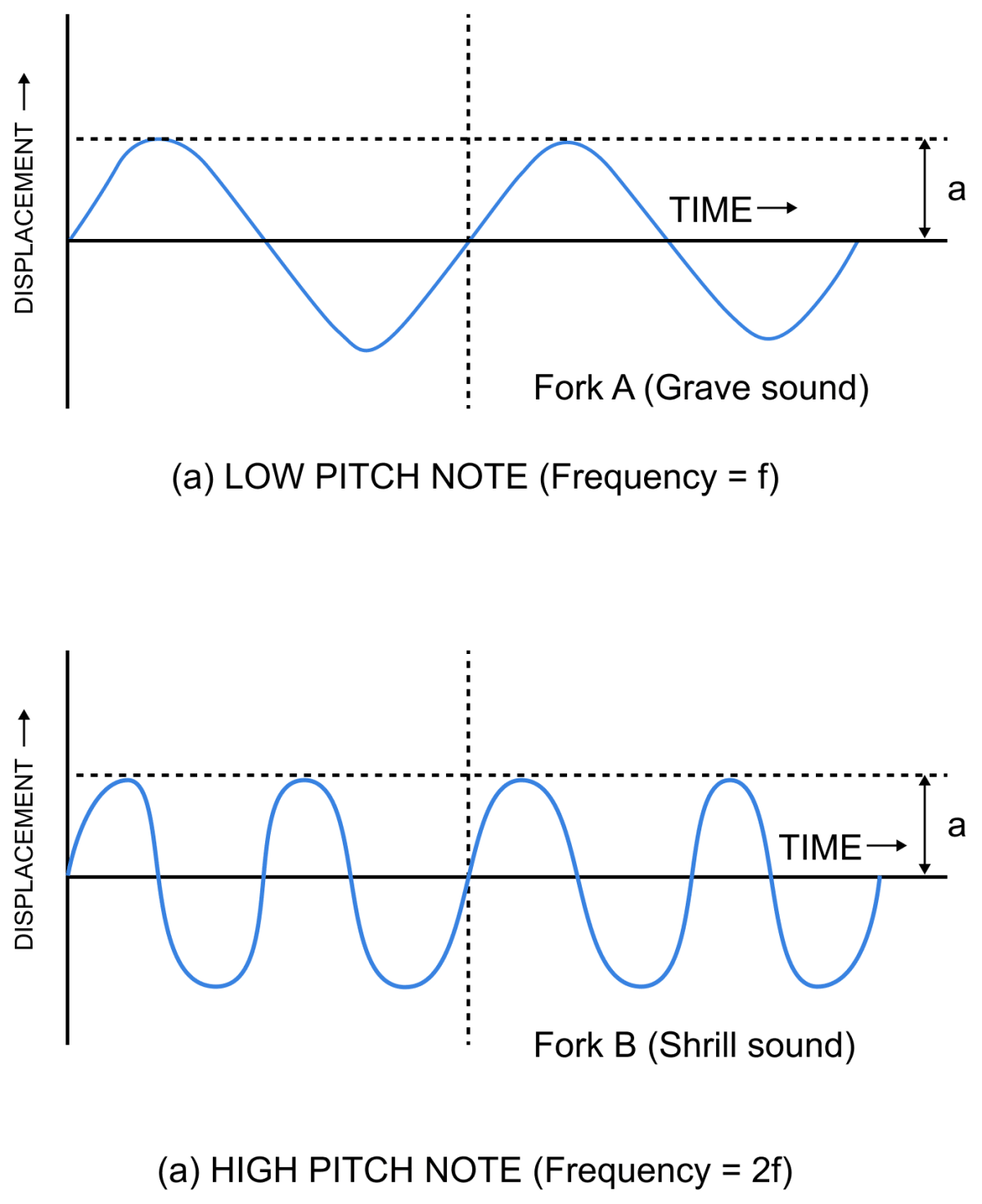#### Question 20

How is it possible to detect the filling of a bottle under a water tap by hearing the sound at a distance?

It is possible to detect the filling of a bottle under a water tap by hearing the sound at a distance due to the pitch of the sound.

As the water level in a bottle kept under a water tap rises, the length of air column decreases, so the frequency of sound produced increases.

As frequency increases the pitch becomes shriller.

Hence, by hearing the sound from a distance, one can get an idea of water level in the pitch.

#### Question 21

The frequencies of notes given by flute, guitar and trumpet are respectively 400 Hz, 200 Hz and 500 Hz. Which one of these has the highest pitch?

As we know, pitch of a sound depends on its frequency and more the frequency of note, higher is it's pitch and less the frequency of note, lower is it's pitch.

We can see from the given data that the frequency of trumpet is highest.

Hence, the pitch of trumpet will be highest.

#### Question 22

Complete the following sentences :

(a) The pitch of sound increases, if it's frequency ........ .

(b) If the amplitude of the sound is halved, it's loudness becomes ........ .

(a) The pitch of sound increases, if it's frequency increases.

(b) If the amplitude of the sound is halved, it's loudness becomes one-fourth.

#### Question 23

The diagram below shows three different modes of vibration P, Q and R of the same string of a given length.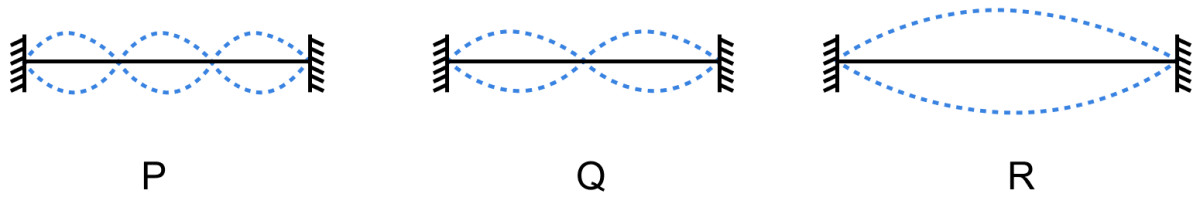(a) Which vibration will produce a louder sound and why?

(b) Which vibration will produce sound of maximum shrillness (or pitch) and why?

(c) What is the ratio of the wavelength of vibrations P and R?

(a) The vibration which produces loudest sound is R, because it's amplitude is maximum.

(b) The vibration which produces maximum shrillness (or pitch) is P, because it's frequency is maximum.

(c) Let l be the length of the string.

Wavelength of P (λp) = $\dfrac{2l}{3}$

Wavelength of R (λR) = 2l

Therefore, ratio of λp : λR =

$= \dfrac{2l}{3 \times 2l} \\[0.5em] = \dfrac{1}{3} \\[0.5em]$

Hence,

λP : λR = 1 : 3

#### Question 24

Name the characteristic which enables one to distinguish the sound of two musical instruments even if they are of the same pitch and same loudness.

The characteristic which enables one to distinguish the sound of two musical instruments even if they are of the same pitch and same loudness is quality.

#### Question 25

How does the two sounds of the same loudness and same pitch produced by different instruments differ? Draw diagrams to illustrate your answer.

Quality (or timbre) of a sound is the characteristic which distinguishes the two sounds of same loudness and same pitch, but emitted by two different instruments because of change in their wave form.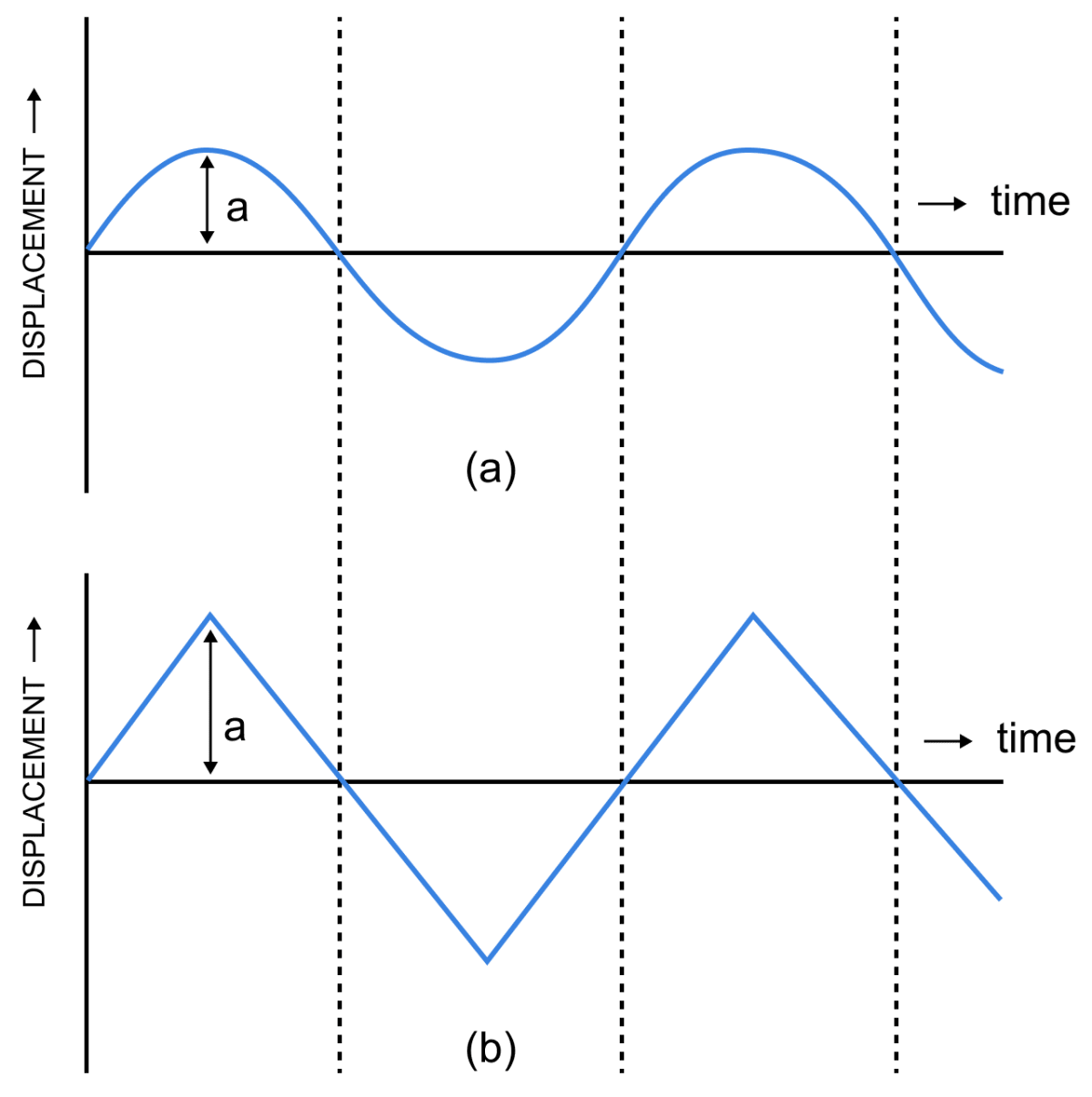The figure above shows the wave forms of two sounds of same loudness (i.e., same amplitude) and same pitch (i.e., same frequency), but emitted by two different sources.

They produce different sensation in our ears because they differ in wave form, one is a sine wave while other is a triangular wave.

#### Question 26

Two identical guitars are played by two persons to give notes of the same loudness and pitch. Will they differ in quality? Give a reason for your answer.

When two identical guitars are played by two persons to give notes of the same loudness and pitch then they will not differ in quality as the identical guitars will produce identical wave forms.

Quality of a musical instrument depends on the number of subsidiary notes and their relative amplitudes present in it along with the principal note.

As same instruments produce same principal and subsidiary notes. Hence, the quality of identical guitars will be same.

#### Question 27

Two musical notes of the same pitch and same loudness are played on two different instruments. Their wave patterns are as shown in figure below.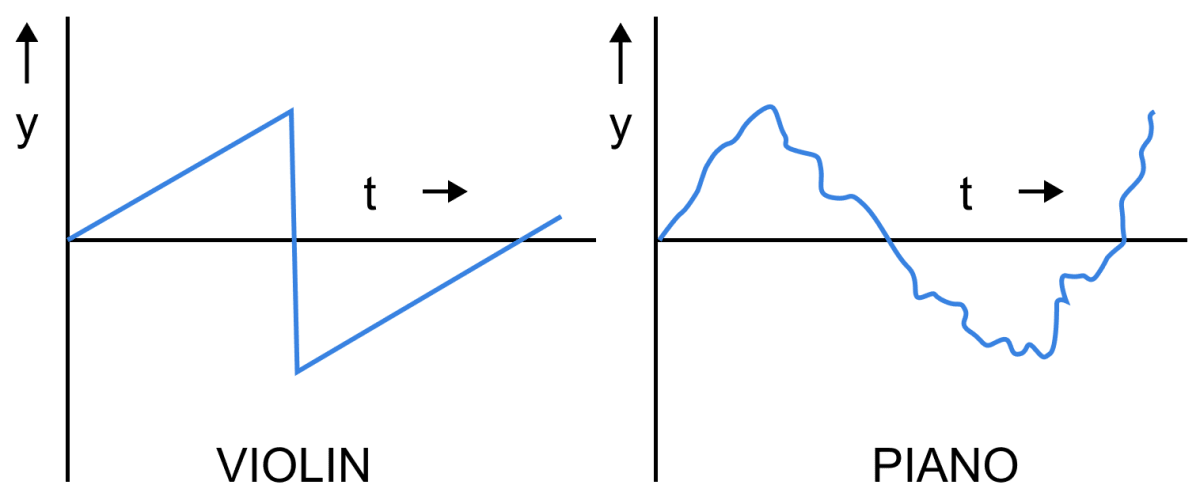Explain why are the wave patterns different.

Different instruments produce different subsidiary notes. A note played on piano has a large number of subsidiary notes, while the same note played on violin does not contain so many subsidiary notes.

Hence, they have different waveforms.

#### Question 28

Which characteristic of sound makes it possible to recognize a person by his voice without seeing him?

We generally recognize a person by his voice without seeing him because the vibrations produced by the vocal chord of each person have a characteristic wave form which is different for different persons.

#### Question 29

State the factor that determines —

1. the pitch of a note,
2. the loudness of the sound heard, and
3. the quality of the note.

1. the pitch of a note — Frequency
2. the loudness of the sound heard — Amplitude
3. the quality of the note — Wave Forms.

#### Question 30

Name the characteristic of sound affected due to a change in it's (i) amplitude, (ii) wave form, and (iii) frequency.

(i) The characteristic of the sound affected due to a change in its amplitude is Loudness.

(ii) The characteristic of the sound affected due to a change in it's wave form is Quality.

(iii) The characteristic of the sound affected due to a change in it's frequency is Pitch.

#### Question 31

The sketches I to IV in figure below show sound waves, all formed in the same time interval.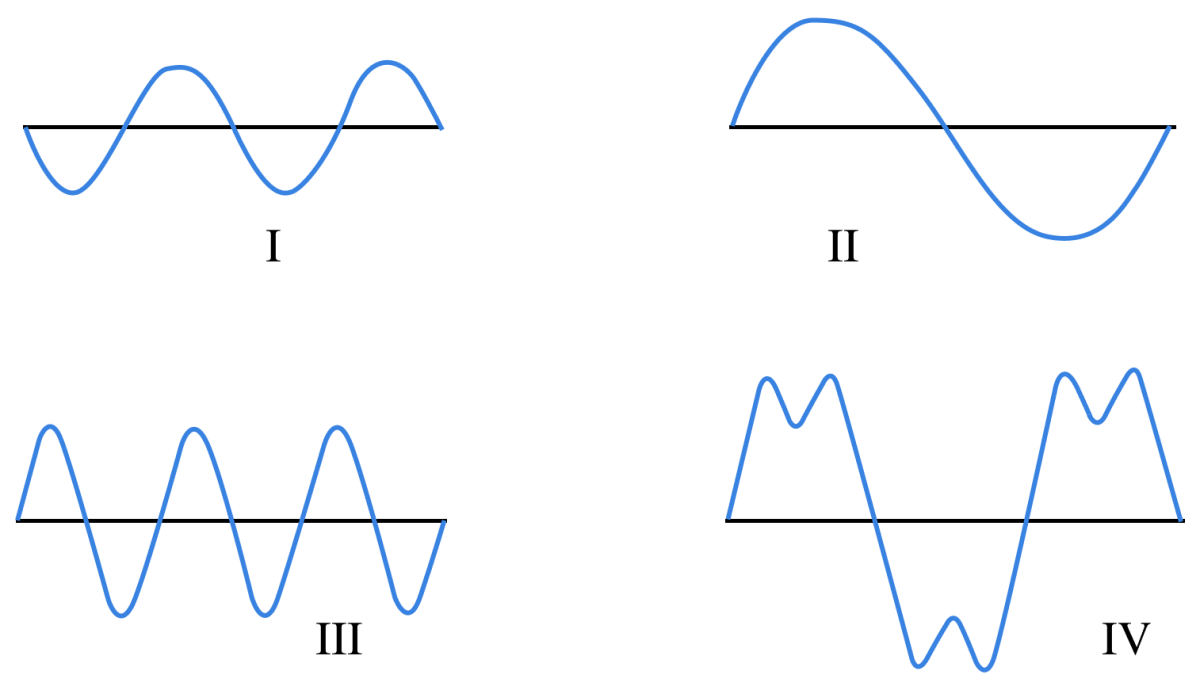Which diagram shows

(i) a note from a musical instrument,

(ii) a soft (or feeble) note,

(iii) a bass (or low frequency) note.

(i) Note from a musical instrument is shown by figure IV as the sketch has subsidiary notes and all musical instruments produce subsidiary notes.

(ii) Soft (or feeble) note is shown by figure I, as we can observe from the wave form that it has the lowest amplitude.

(iii) Bass (or low frequency) note is shown by Figure II, as we can observe from the wave form that it has the lowest frequency.

#### Question 32

Figure below shows the wave patterns of three sounds A, B and C. Name the characteristic of sound which is same between (i) A and B, (ii) B and C, and (iii) C and A.(i) When we observe the figure shown, we find that both A and B have same amplitude and wave form.

Hence, the characteristics of sound which are same in both A and B are loudness and quality.

(ii) When we observe the figure shown, we find that in B and C, none of the characteristics are same.

(iii) When we observe the figure shown, we find that in both C and A, frequency is same. Hence, pitch is same.

#### Question 33

A microphone is connected to the Y-input of a C.R.O. Three different sounds are made in turn in front of the microphone. Their traces (a), (b) and (c) produced on the screen are shown in figure.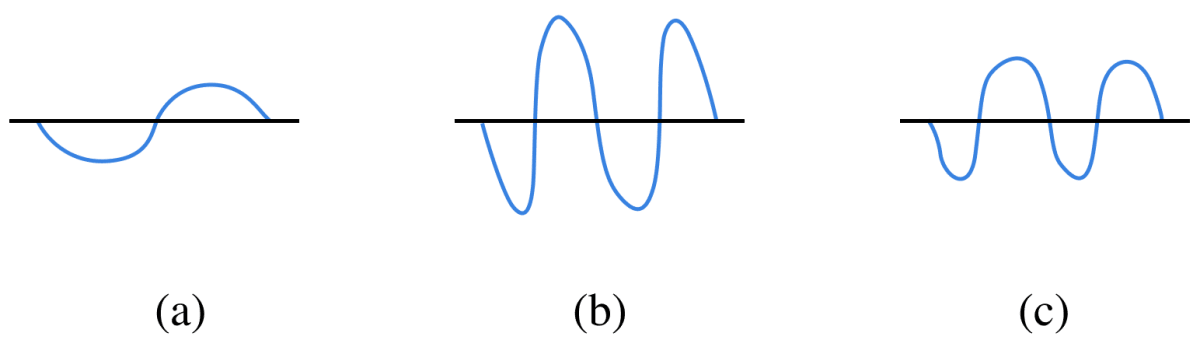(i) Which trace is due to the loudest sound? Give reason for your answer.

(ii) Which trace is due to the sound with the lowest pitch? Explain your answer.

(i) The trace with loudest sound is b, since amplitude is largest.

(ii) The trace representing the sound with lowest pitch is a, since frequency is lowest.

#### Question 34

In what respect does the wave pattern of a noise and music differ? Draw diagram to explain your answer.

Noise is a sound produced by an irregular succession of disturbances. It is usually a discontinuous sound. Hence, the wave pattern is also irregular.

Music is a pleasant, continuous and uniform sound produced by the regular and periodic vibrations. Hence, the wave form is regular.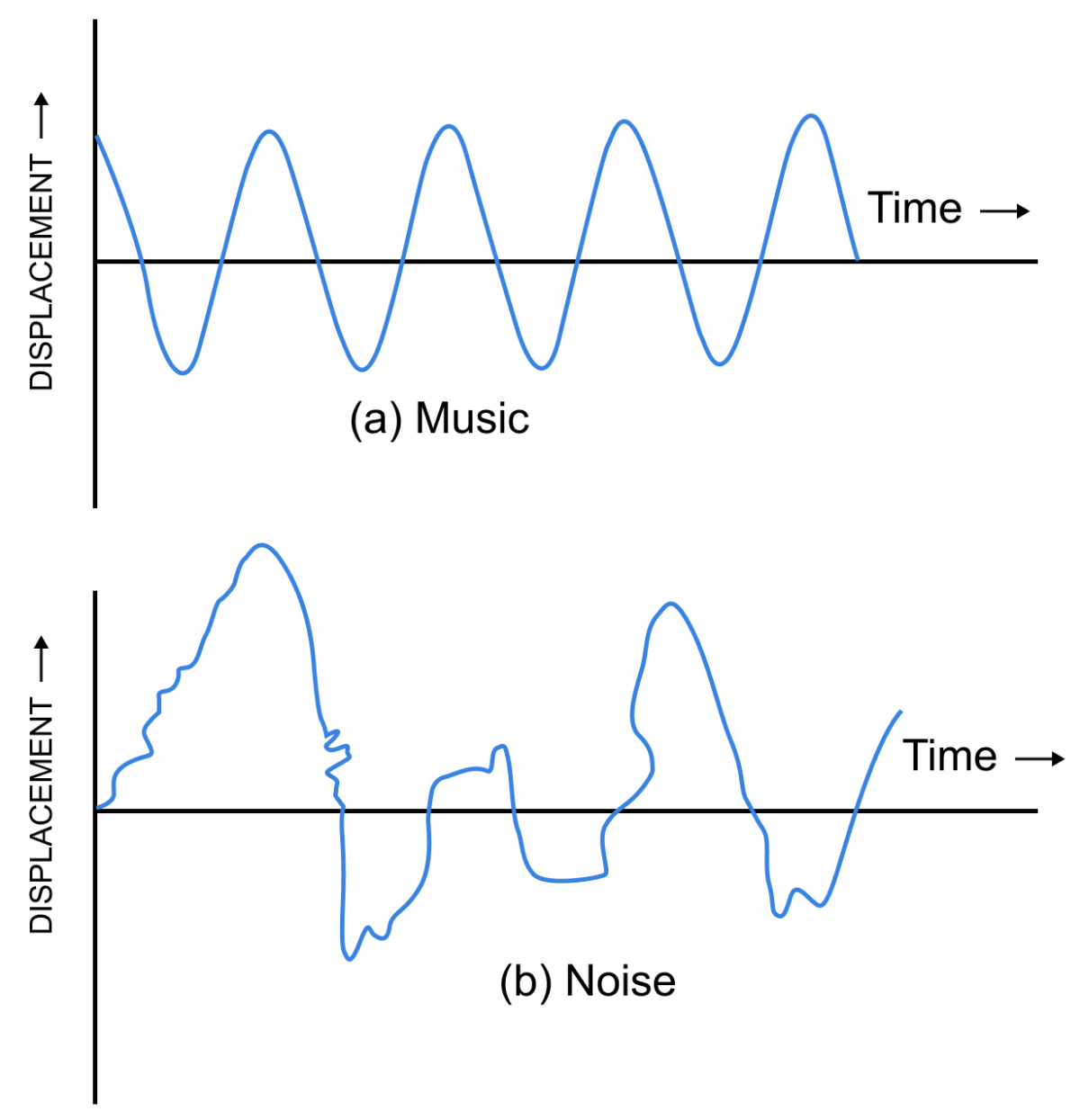#### Question 35

State one difference between a musical note and noise.

Musical noteNoise
It is regular, smooth and pleasant to the ears.It is harsh, discordant and unpleasant to the ears.

## Multiple Choice Type

#### Question 1

By reducing the amplitude of the sound wave, its —

1. pitch increases
2. loudness decreases ✓
3. loudness increases
4. pitch decreases

Loudness is proportional to the square of the amplitude

Loudness ∝ (amplitude) 2

So, when a body vibrates with a lesser amplitude, it sends forth a smaller amount of energy.

Hence, the energy received by the ear drum is also small, so the loudness of the sound decreases.

#### Question 2

Two sounds of same loudness and same pitch produced by two different instruments differ in their

1. amplitudes
2. frequencies
3. wave forms ✓
4. all the above

Two sounds of same loudness and same pitch produced by the two different instruments differ in their waveforms.

#### Question 3

Two sounds A and B are of same amplitudes, same waveforms but of frequencies f and 2f respectively. Then :

1. B differ in quality from A
2. B is grave, A is shrill
3. B is shrill, A is grave ✓
4. B is louder than A